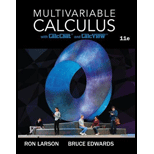# Moment of Inertia In Exercises 29 and 30, use cylindrical coordinates to verify the given moment of inertia of the solid of uniform density. Right circular cylinder: I z = 3 2 m a 2 r = 2 a sin θ , 0 ≤ z ≤ h (Use a computer algebra system to evaluate the triple integral.)### Multivariable Calculus

11th Edition
Ron Larson + 1 other
Publisher: Cengage Learning
ISBN: 9781337275378

#### Solutions

Chapter
Section### Multivariable Calculus

11th Edition
Ron Larson + 1 other
Publisher: Cengage Learning
ISBN: 9781337275378
Chapter 14.7, Problem 30E
Textbook Problem
1 views

## Moment of Inertia In Exercises 29 and 30, use cylindrical coordinates to verify the given moment of inertia of the solid of uniform density.Right circular cylinder: I z = 3 2 m a 2 r = 2 a sin θ ,       0 ≤ z ≤ h (Use a computer algebra system to evaluate the triple integral.)

This textbook solution is under construction.

### Still sussing out bartleby?

Check out a sample textbook solution.

See a sample solution

#### The Solution to Your Study Problems

Bartleby provides explanations to thousands of textbook problems written by our experts, many with advanced degrees!

Get Started